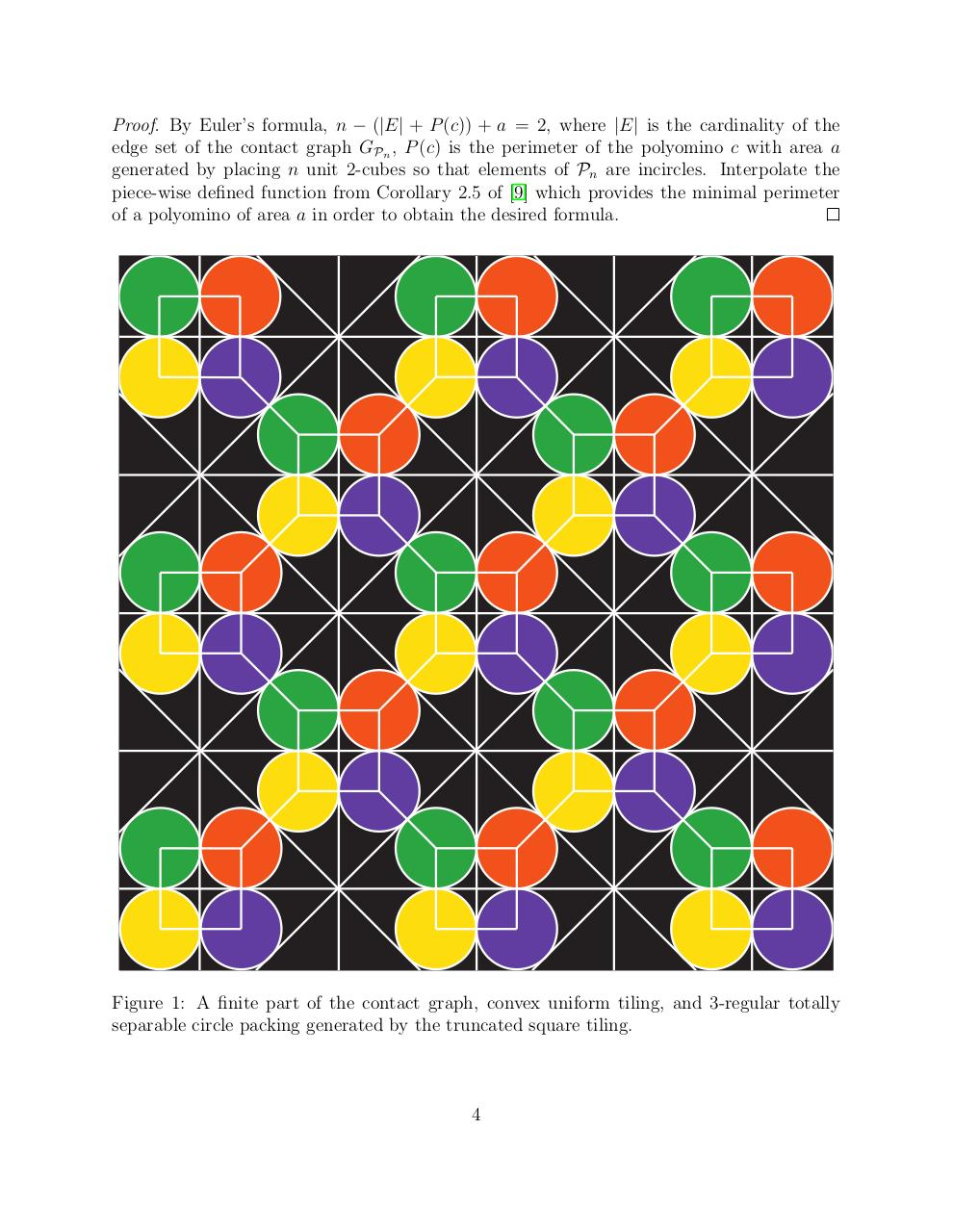# Regular Totally Separable Sphere Packings arXiv.pdfPage 1 2 3 4 5 6 7 8 9 10

#### Text preview

Proof. By Euler’s formula, n − (|E| + P (c)) + a = 2, where |E| is the cardinality of the
edge set of the contact graph GPn , P (c) is the perimeter of the polyomino c with area a
generated by placing n unit 2-cubes so that elements of Pn are incircles. Interpolate the
piece-wise defined function from Corollary 2.5 of  which provides the minimal perimeter
of a polyomino of area a in order to obtain the desired formula.

Figure 1: A finite part of the contact graph, convex uniform tiling, and 3-regular totally
separable circle packing generated by the truncated square tiling.

4